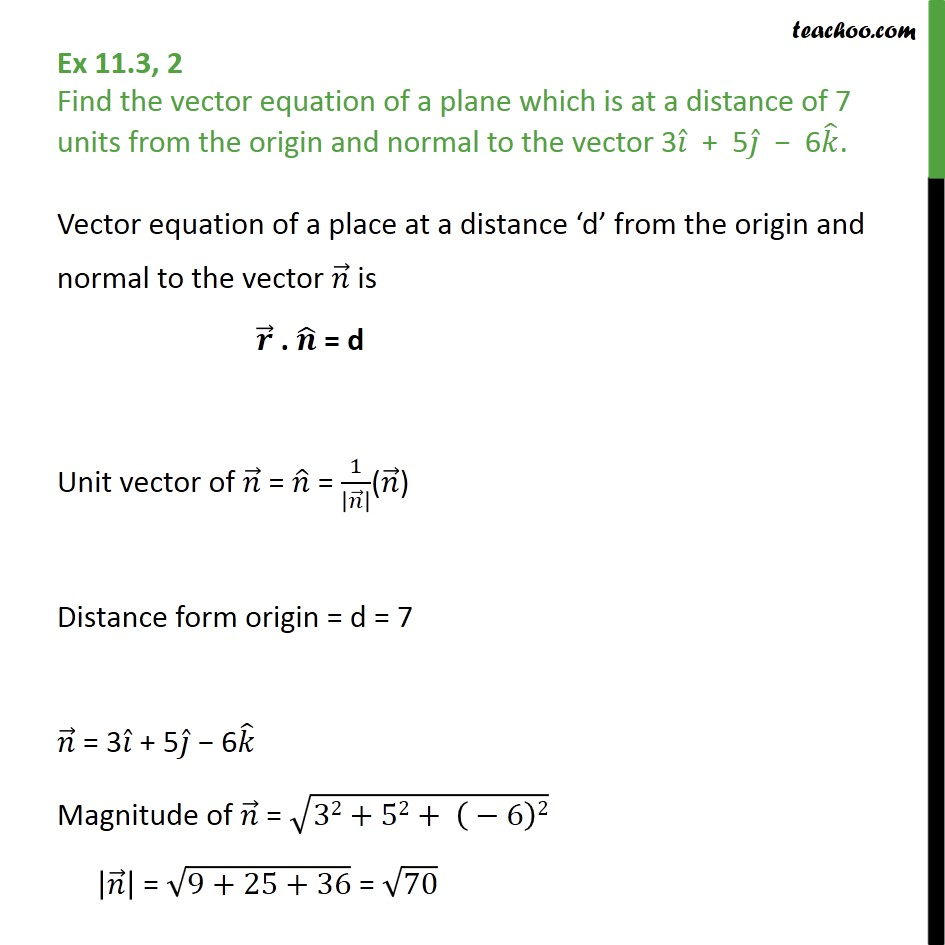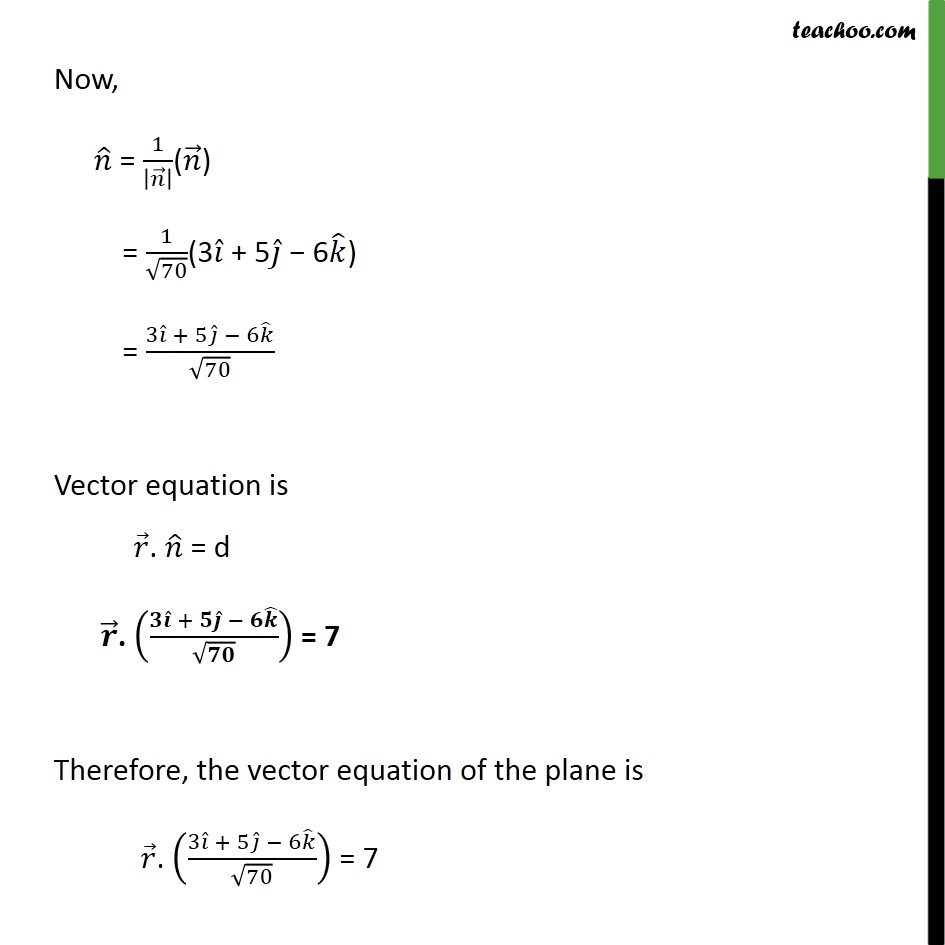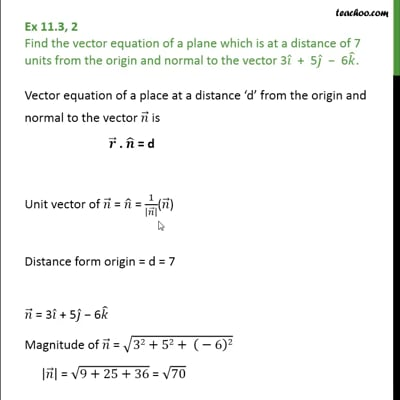Ex 11.3

Chapter 11 Class 12 Three Dimensional Geometry
Serial order wiseThis video is only available for Teachoo black users

Get live Maths 1-on-1 Classs - Class 6 to 12

### Transcript

Ex 11.3, 2 Find the vector equation of a plane which is at a distance of 7 units from the origin and normal to the vector 3 + 5 6 . Vector equation of a place at a distance d from the origin and normal to the vector is . = d Unit vector of = = 1 ( ) Distance form origin = d = 7 = 3 + 5 6 Magnitude of = 32+52+ 6 2 = 9+25+36 = 70 Now, = 1 ( ) = 1 70 (3 + 5 6 ) = 3 + 5 6 70 Vector equation is . = d . + = 7 Therefore, the vector equation of the plane is . 3 + 5 6 70 = 7